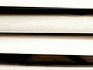PESO Y LONGITUD Weight and LengthLOS CURSOS DE INGLES GRATIS PREFERIDOS POR LOS HISPANOHABLANTES

 Audio

 Weight Peso
 Sistema Británico Sistema Métrico Decimal
 1 ounce (oz) = 28.35 grams (g) 16 ounces = 1 pound (lb) = 0.454 kilogram (kg) 14 pounds = 1 stone (st) = 6.356 kilograms (kg)
 Sistema en EE UU Sistema Métrico Decimal
 1 ounce (oz.) = 31.103 grams (g) 16 ounces = 1 pound (lb.) = 0.373 kilogram (kg) 2000 pounds = 1 ton (t.) = 0.907 metric ton (m.t.) (kg)
 Examples: Our baby Mike is weighing 8 lb 6 oz (eight pounds six ounces). To make this cake you need 550g (five hundred and fifty grams) of chocolate.
 Length Longitud
 Sistema Británico y en EE UU Sistema Métrico Decimal
 1 inch (in) = 25.4 millimetres (mm) 12 inches 1 ounce (ft) = 30.48 centimetres (cm) 3 feet = 1 yard (yd) = 0.914 metre (m) 1760 yards = 1 mile = 1.609 kilometres (km)
 Examples: Height: 15 ft 6 in (fifteen foot six or fifteen feet six). The new inn is 50 yds (fifty yards) from the town. The bus was doing 60 mph (sixty miles per hour). The sitting area is 20' x 10' 5" (twenty foot by ten foot five or twenty feet by ten feet five).